# The Universe of Time-Series Forecasting Techniques: A Primer

Time-series forecasting is one of the most talked-about topics in data science. Not surprisingly, there is a rich forecasting toolbox with many different options to choose from for data scientists. The possibilities are so many that they often leave data scientists being overwhelmed, puzzled, and sometimes outright confused.

More often than not, these techniques are closely related to each other. Limitations in one technique most likely led to the development of another. As we shall see below, for example, all techniques in the ARIMA family (e.g., AR, MA, SARIMA, SARIMAX) may look different, but in reality, they are simply a variation of each other.

So the motivation behind writing this article is to put them all together so that it’s easy to compare similarities and differences. I am hoping, at the end of the article, the readers are less confused and have at least a superficial understanding of when (not) to use a specific technique in practice.

I will simplify things as much as possible, focusing more on breadth rather than depth, presenting them in increasing order of complexity. But to be clear, model complexity alone doesn’t guarantee a better prediction; to get better results, there is much more than just building sophisticated models.

# 1. Benchmark forecasting

These models are known as the so-called “benchmark” or “baseline” forecasting.

As you will see below, these techniques are rarely applied in practice, but they help build forecasting intuition upon which to add additional layers of complexity.

1.1 Naive Forecast:

In Naive forecast, the future value is assumed to be equal to the past value. So the sales volume of a particular product on Wednesday would be similar to Tuesday’s sales.

Naive forecast acts much like a null hypothesis against which to compare an alternative hypothesis — sales revenue will be different tomorrow because of such and such reasons.

1.2 Seasonal Naive:

Seasonal naive, as the name suggests, factors in seasonality in its forecast. So in a way, it is an improvement over Naive forecast. The revenue forecast for December would be equal to the previous year’s December revenue because holidays are factored in.

Again, it still works like a null hypothesis but considers seasonality as its key improvement over Naive forecast.

1.3 Mean Model

Naive forecast takes one past value and uses it as a predicted value. The mean model, in contrast, takes all the past observations, makes an average, and uses this Average as the forecast value.

If data is randomly distributed, without clear patterns and trends (also known as the white noise), a mean model works as a better benchmark than a naive model.

1.4 Drift model

The drift model is yet another variation of Naive forecast, with an obvious improvement. As in Naive, it takes the last observation, but then adjusts the observation based on variation in past values.

Forecast value = past observation +/- average change in past observations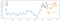Mean and drift model with respect to training and testing data (source: author)

1.5 Linear Trend

The mean model described above is a horizontal, constant line that doesn’t change over time because it works on training data without a trend. However, if a trend is detected, a linear model provides a better forecast value than a Mean model.

Forecasting using Linear Trend in practice is actually the line of best fit (i.e., regression line) of the following form:

Y(t) = alpha + beta*t

An RSME or R2 value determines how good the fitted line is for prediction.

1.6 Random Walk

In this case, the forecast value “walks” a random step ahead from its current value (similar to Brownian Motion). Like a walking toddler, the next step can be in any random direction but isn’t too far from where the last step was.

Y(t+1)=Y(t) + noise(t)

The stock price on Wednesday will likely be close to Tuesday’s closing price, so a Random Walk provides a reasonable guestimate. But it’s not suitable to predict too many time-steps ahead, because, well, each step is random.

1.7 Geometric Random Walk

In Geometric Random Walk, the forecast for the next value will be equal to the last value plus a constant change (e.g., a percentage monthly increase in revenue).

Ŷ(t) = Y(t-1) + α

It’s also called the “random-walk-with-growth model.” Stock prices in the long-term follow somewhat a Geometric Random Walk model.

# 2. Exponential smoothing

If decomposed, a time series will disaggregate into 3 components: trend, seasonality, and white noise (i.e., random data points). For forecasting purposes, we can predict the predictable components (i.e., trend and seasonality), and not the unpredictable terms which occur in a random fashion. Exponential smoothing can handle this kind of variability within a series by smoothing out white noise.

A Moving Average can smooth training data, but it does so by taking an average of past values and by weighting them equally. On the other hand, in Exponential Smoothing, the past observations are weighted in an exponentially decreasing order. Meaning, most recent observations are given higher weights than far-away values.

Exponential smoothing has few variants for different data types.

2.1 Simple Exponential Smoothing

A Simple Exponential Smoothing is used for data without a clear trend or seasonality.

2.2 Holt’s linear trend

Holt’s method is similar to Simple Exponential Smoothing but used for data with a clear trend.

2.3 Holt-Winter Exponential Smoothing

Holt-Winter method is for a series that has both a trend and seasonality, meaning, and it’s a combination of the previous two techniques. Holt-Winter is applied to a stationary series, and smoothing is controlled by the weighting parameter alpha (0~1).

# 3. ARIMA Family

I call them the ARIMA family because they are a suite of techniques closely related to each other.

3.1 Autoregressive (AR)

Before going into autoregression, let’s refresh memory on linear regression with a dependent variable and one or more independent variables:

Sales = f(customer income, promotion)

Autoregression is also a kind of linear regression, but in this case, independent variables are the past values of the series itself.

Sales in Wed = f(sales in Tues, Mon, Sun, Sat …etc)

Autoregression is represented by AR(p), where p determines how many past values are used to predict the future.

3.2 Moving average (MA)

A Moving Average is calculated by taking a mean of any number of past observations. These mean values are then used to forecast future values.

Not just forecasting, Moving Average is a useful tool for understanding general patterns and trends in data, especially in a noisy series.

Moving Average is represented as MA(q), where q is the number of past observations.

3.3 ARMA

As the name suggests, ARMA is a combination of AR and MA processes described above:

Y = c + X + AR term + MA term

To put it in real-world terms:

Today’s value = mean + noise + yesterday’s value + yesterday’s noise.

3.4 ARIMA

Autoregressive Integrated Moving Average (ARIMA) is arguably the most popular and widely used statistical technique for forecasting. As the name suggests, ARIMA has 3 components: a) an Autoregressive component to model the relationship between the series and it’s lagged values; b) a Moving Average component that predicts future value as a function of lagged forecast errors; and c) an Integrated component that makes the series stationary.

Making a time series stationary means removing the trend component. It is done in a number of ways, one is by taking differences between the data, and it’s lagged values.

ARIMA model — represented as ARIMA(p, q, d) — takes the following parameters:

• p that defines the number of lags;
• d that specifies the number of differences used; and
• q that defines the size of moving average window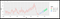Forecasting new family homes sales in the US using ARIMA (source: author)

3.5 SARIMA

SARIMA is nothing but Seasonal ARIMA.

ARIMA is great for predicting a series with trends, but SARIMA is better for predicting the seasonal component of a series. Mathematically it is represented as:

SARIMA(p, d, q)(P, D, Q)m

Where, the (p, d, q) component comes from ARIMA, and (P, D, Q)m component makes it a SARIMA, where:

• P: seasonal AR order
• Q: seasonal difference order
• D: seasonal MA order
• m: number of time steps in a seasonal cycle (e.g., 12 for a year, 4 for a quarterly cycle)

3.6 ARIMAX

So far, we have talked about forecasting a series with a single variable and using its past observations only. It’s like forecasting future population solely based on historical population.

Future population = f(past population)

But we know that the past population is one of many factors to determine future population — such as birth rate, mortality, education, income, etc. These factors are called eXogenous factors or co-variates:

Future population = f(past population, birth, mortality, income ….. etc.)

So ARIMAX is a multivariate version of ARIMA that makes forecasts based on lagged values of the series itself and lagged values of exogenous variables.

4.1 Regression

Regression, a fairly well-researched technique, predicts a dependent variable as a function of one or more independent variables. The independent variable(s), in this case, must have a linear relationship with the variable being predicted. For example, if revenue from product sales is to be predicted, product price can be an independent variable because prices directly affect how many units will be sold:

Revenue = f(product price)

The above model is called Simple Linear Regression because it has only one predictor. A variation of it — the Multiple Linear Regression — takes more than one predictor. Again, each predictor needs to be in a linear relationship with the variable being predicted:

Units sold = f(product price, customer income)

4.2 Fast Fourier Transform (FFT)

(FFT) was originally developed for application in signal processing, but eventually found it’s way into time series analysis and forecasting.

There are quite a few applications of FFT forecasting — product demand forecasting, financial forecasting, inbound call prediction are few of them.

FFT technique is not as widely applied in data science forecasting as in other fields, so available resources and software packages are limited (but see `numpy.fft.fft `and a worked-out example).

4.3 Vector Autoregressive model (VAR)

VAR is yet another multivariate forecasting model with exogenous variables. In this model, each variable is forecasted using its own past (lag) values as well as the lag values of exogenous factors. It takes only one parameter, p:

VAR(p), where p is the number of lags

There are some theoretical differences between ARIMAX and VAR, but it requires a long discussion, which I’ll cover in a future post.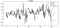GDP is forecasted in VAR with several independent variables (source: author)

4.4 ARCH

Autoregressive Conditionally Heteroscedastic (ARCH) — a mouthful name, but a different kind of model applied in forecasting heteroscedastic time series.

It is most commonly used in econometric modeling of volatile, high variance time series data. ARCH is formalized with one parameter:

ARCH(m), where variance at time t is conditional on past m observations

4.5 Deep Learning/RNN/LSTM

Long Short-Term Memory (LSTM), a type of recurrent neural network commonly used in deep learning, is also a useful tool for time series forecasting.

The key strength of LSTM is that it can be used both for univariate and multivariate predictions.

Most deep learning frameworks, `PyTorch`, `Tensorflow` and `Keras`have built-in capabilities to run time series LSTM. Check out this `Tensorflow` implementation of LSTM.

4.6 Panel data models

A panel data is a multi-dimensional data of observations measured repeatedly over time. In other words, it’s a dataset where multiple variables are measured, over time, on the same units — such as individuals, organizations, households, city, country.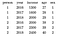Example of a panel data of 3 variables, 2 individuals measured over 3 years time (source: author)

Three main types of panel data models (i.e., estimators) are used in time series forecasting: Pooled OLS, Random Effects Model, and Fixed Effects Model.

4.7 System dynamics modeling (SD)

System Dynamics is a methodological approach for complex systems modeling, where a change in one element leads to a change in others.

SD is widely applied in healthcare, epidemiology, transportation, business management, and revenue forecasting. The most famous of all is arguably modeling Limits to Growth by the Club of Rome.

A System Dynamic model represents a complex system in terms of stocks & flows and their interactions via feedback loops to predict the behavior of the system. Let’s say a bank account has a “stock” of \$100. Every month \$20 is deposited (represented by Flow 1), and an amount of \$15/month is withdrawn (Flow 2). In this simple case, a change in Flow 1 will cause a change in Stock 1 and Flow 2. So if we know how Flow 1 will evolve into the future, we can forecast both Stock 1 and Flow 2.

4.8 Agent-based Modeling (ABM)

Similar to SD, Agent-based models are computational models for simulating actions or movements of individuals (called “agents”) and their interactions. It has a suite of tools and techniques used in modeling complex social, economic, and environmental systems.

ABMs have been frequently used in forecasting stock market movement, infectious disease prediction, species distribution modeling, and modeling a wide range of economic and environmental issues.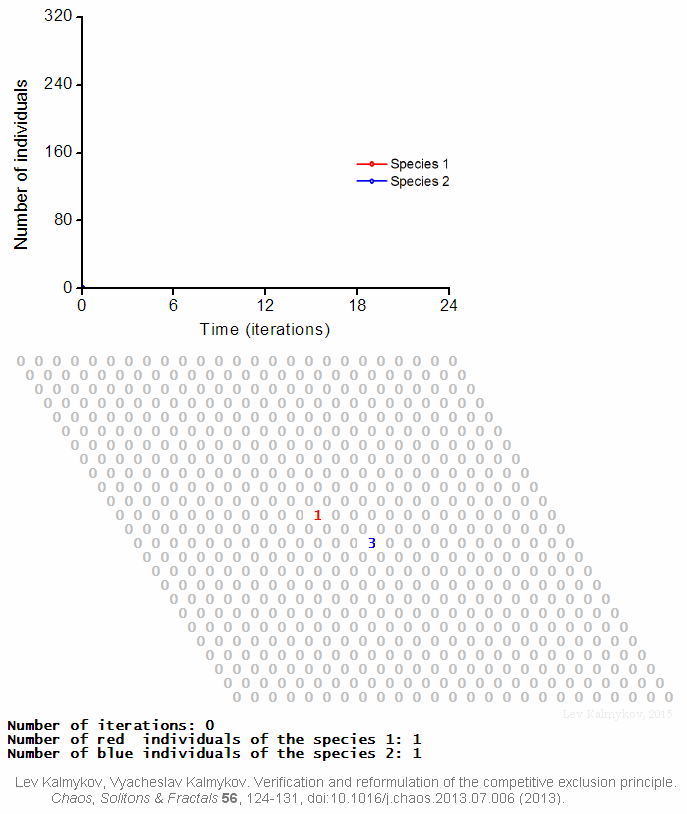Agent-Based modeling for forecasting. By Lev Kalmykov — CC BY-SA 4.0, https://commons.wikimedia.org/w/index.php?curid=41287917

# Final thoughts

Time series forecasting has a rich set of machine learning tools and techniques. That means it’s easy to get lost when choosing a particular technique for forecasting. In this post, I’ve outlined key characteristics of each method in a way to reveal their commonalities while demonstrating the key differences. As I said, these techniques aren’t isolated, rather interrelated, and one exists because of limitations in another.

## Towards AI — Multidisciplinary Science Journal

### By Towards AI — Multidisciplinary Science Journal

Towards AI publishes the best of tech, science, and engineering. Subscribe with us to receive our newsletter right on your inbox. Take a look

Written by

Written by

## Mahbubul Alam